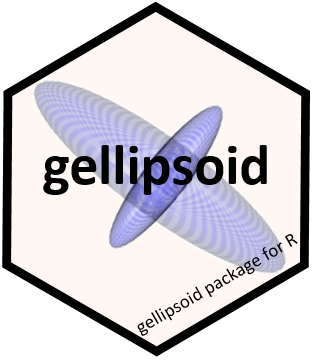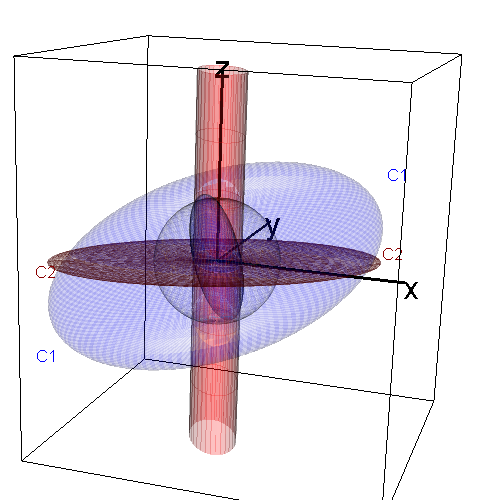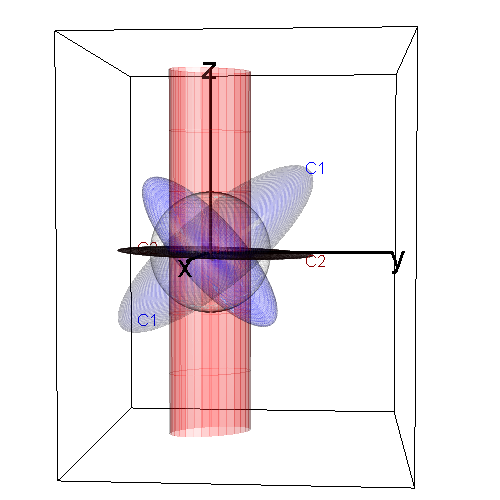# gellipsoid: Generalized EllipsoidsThe gellipsoid package extends the class of geometric ellipsoids to “generalized ellipsoids”, which allow degenerate ellipsoids that are flat and/or unbounded. Thus, ellipsoids can be naturally defined to include lines, hyperplanes, points, cylinders, etc. The methods can be used to represent generalized ellipsoids in a-dimensional space, with plots in up to 3D.

The goal is to be able to think about, visualize, and compute a linear transformation of an ellipsoid with central matrixor its inversewhich apply equally to unbounded and/or degenerate ellipsoids.

The implementation uses arepresentation, based on the singular value decomposition (SVD) of an ellipsoid-generating matrix,, whereis square orthogonal andis diagonal.

For the usual, “proper” ellipsoids,is positive-definite so all elements ofare positive. In generalized ellipsoids,is extended to non-negative real numbers, i.e.  its elements can be 0, Inf or a positive real.

#### Definitions

A proper ellipsoid incan be defined bywhereis a non-negative definite central matrix, In applications,is typically a variance-covariance matrix A proper ellipsoid is bounded, with a non-empty interior. We call these fat ellipsoids.

A degenerate flat ellipsoid corresponds to one where the central matrixis singular or when there are one or more zero singular values in. In 3D, a generalized ellipsoid that is flat in one dimension () collapses to an ellipse; one that is flat in two dimensions () collapses to a line, and one that is flat in three dimensions collapses to a point.

An unbounded ellipsoid is one that has infinite extent in one or more directions, and is characterized by infinite singular values in. For example, in 3D, an unbounded ellipsoid with one infinite singular value is an infinite cylinder of elliptical cross-section.

## Principal functions

• gell() Constructs a generalized ellipsoid using therepresentation. The inputs can be specified in a variety of ways:

• a non-negative definite variance matrix;
• an inner-product matrix
• a subspace with a given span
• a matrix giving a linear transformation of the unit sphere
• dual() calculates the dual or inverse of a generalized ellipsoid

• gmult() calculates a linear transformation of a generalized ellipsoid

• signature() calculates the signature of a generalized ellipsoid, a vector of length 3 giving the number of positive, zero and infinite singular values in the (U, D) representation.

• ell3d() Plots generalized ellipsoids in 3D using the rgl package

## Installation

You can install the development version of gellipsoid from GitHub with:

# install.packages("devtools")
devtools::install_github("friendly/gellipsoid")

## Example

#### Properties of generalized ellipsoids

The following examples illustrate gell objects and their properties. Each of these may be plotted in 3D using ell3d(). These objects can be specified in a variety of ways, but for these examples the span is simplest.

A unit sphere inhas a central matrix of the identity matrix.

library(gellipsoid)
(zsph <- gell(Sigma = diag(3)))  # a unit sphere in R^3
#> $center #>  0 0 0 #> #>$u
#>      [,1] [,2] [,3]
#> [1,]    0    0    1
#> [2,]    0    1    0
#> [3,]    1    0    0
#>
#> $d #>  1 1 1 #> #> attr(,"class") #>  "gell" signature(zsph) #> pos zero inf #> 3 0 0 isBounded(zsph) #>  TRUE isFlat(zsph) #>  FALSE A plane inis flat in one dimension. (zplane <- gell(span = diag(3)[, 1:2])) # a plane #>$center
#>  0 0 0
#>
#> $u #> [,1] [,2] [,3] #> [1,] 1 0 0 #> [2,] 0 1 0 #> [3,] 0 0 1 #> #>$d
#>  Inf Inf   0
#>
#> attr(,"class")
#>  "gell"
signature(zplane)
#>  pos zero  inf
#>    0    1    2
isBounded(zplane)
#>  FALSE
isFlat(zplane)
#>  TRUE

dual(zplane)  # line orthogonal to that plane
#> $center #>  0 0 0 #> #>$u
#>      [,1] [,2] [,3]
#> [1,]    0    0    1
#> [2,]    0    1    0
#> [3,]    1    0    0
#>
#> $d #>  Inf 0 0 #> #> attr(,"class") #>  "gell" signature(dual(zplane)) #> pos zero inf #> 0 2 1 A hyperplane. Note that the gell object with a center contains more information than the geometric plane. (zhplane <- gell(center = c(0, 0, 2), span = diag(3)[, 1:2])) # a hyperplane #>$center
#>  0 0 2
#>
#> $u #> [,1] [,2] [,3] #> [1,] 1 0 0 #> [2,] 0 1 0 #> [3,] 0 0 1 #> #>$d
#>  Inf Inf   0
#>
#> attr(,"class")
#>  "gell"
signature(zhplane)
#>  pos zero  inf
#>    0    1    2

dual(zhplane)  # orthogonal line through same center
#> $center #>  0 0 2 #> #>$u
#>      [,1] [,2] [,3]
#> [1,]    0    0    1
#> [2,]    0    1    0
#> [3,]    1    0    0
#>
#> $d #>  Inf 0 0 #> #> attr(,"class") #>  "gell" A point: zorigin <- gell(span = cbind(c(0, 0, 0))) signature(zorigin) #> pos zero inf #> 0 3 0 # what is the dual (inverse) of a point? dual(zorigin) #>$center
#>  0 0 0
#>
#> $u #> [,1] [,2] [,3] #> [1,] 0 0 1 #> [2,] 0 1 0 #> [3,] 1 0 0 #> #>$d
#>  Inf Inf Inf
#>
#> attr(,"class")
#>  "gell"

signature(dual(zorigin))
#>  pos zero  inf
#>    0    0    3

#### Drawing generalized ellipsoids

The following figure shows views of two generalized ellipsoids.(blue) determines a proper, fat ellipsoid; it’s inversealso generates a proper ellipsoid.(red) determines an improper, flat ellipsoid, whose inverseis an unbounded cylinder of elliptical cross-section.is the projection ofonto the plane where. The scale of these ellipsoids is defined by the gray unit sphere.This figure illustrates the orthogonality of eachand its dual,.Friendly, M., Monette, G. and Fox, J. (2013). Elliptical Insights: Understanding Statistical Methods through Elliptical Geometry. Statistical Science, 28(1), 1-39. https://arxiv.org/abs/1302.4881.

Friendly, M. (2013). Supplementary materials for “Elliptical Insights …”, https://www.datavis.ca/papers/ellipses/